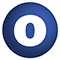# RDDHistogramMatching

### Related Doc: package matching

#### object RDDHistogramMatching

Linear Supertypes
AnyRef, Any
Ordering
1. Alphabetic
2. By Inheritance
Inherited
1. RDDHistogramMatching
2. AnyRef
3. Any
1. Hide All
2. Show All
Visibility
1. Public
2. All

### Value Members

1. #### final def !=(arg0: Any): Boolean

Definition Classes
AnyRef → Any
2. #### final def ##(): Int

Definition Classes
AnyRef → Any
3. #### final def ==(arg0: Any): Boolean

Definition Classes
AnyRef → Any
4. #### final def asInstanceOf[T0]: T0

Definition Classes
Any
5. #### def clone(): AnyRef

Attributes
protected[java.lang]
Definition Classes
AnyRef
Annotations
@throws( ... )
6. #### final def eq(arg0: AnyRef): Boolean

Definition Classes
AnyRef
7. #### def equals(arg0: Any): Boolean

Definition Classes
AnyRef → Any
8. #### def finalize(): Unit

Attributes
protected[java.lang]
Definition Classes
AnyRef
Annotations
@throws( classOf[java.lang.Throwable] )
9. #### final def getClass(): Class[_]

Definition Classes
AnyRef → Any
10. #### def hashCode(): Int

Definition Classes
AnyRef → Any
11. #### final def isInstanceOf[T0]: Boolean

Definition Classes
Any
12. #### def multiband[T <: AnyVal, K, V](rdd: RDD[(K, V)], targetHistograms: Seq[Histogram[T]])(implicit arg0: (V) ⇒ MultibandTile): RDD[(K, MultibandTile)]

Given an RDD of key-MultibandTile pairs and a sequence of target histograms (of the bands of the tiles), this function produces an RDD of key-MultibandTile pairs where the histograms of the bands of the result tiles have been matched to the respective target histograms.

Given an RDD of key-MultibandTile pairs and a sequence of target histograms (of the bands of the tiles), this function produces an RDD of key-MultibandTile pairs where the histograms of the bands of the result tiles have been matched to the respective target histograms.

rdd

The key-MultibandTile pairs

targetHistograms

The histograms that the bands of the the tiles should be matched to

returns

An RDD key-MultibandTile pairs where the bands of the histograms have been matched

13. #### def multiband[T1 <: AnyVal, T2 <: AnyVal, K, V](rdd: RDD[(K, V)], sourceHistograms: Seq[Histogram[T1]], targetHistograms: Seq[Histogram[T2]])(implicit arg0: (V) ⇒ MultibandTile): RDD[(K, MultibandTile)]

Given an RDD of key-MultibandTile pairs, a sequence of source histograms (ostensibly those of the bands of the tiles in the RDD), and a sequence of target histograms (of the bands of the tiles), this function produces an RDD of key-MultibandTile pairs where the histograms of the bands of the result tiles have been matched to the respective target histograms.

Given an RDD of key-MultibandTile pairs, a sequence of source histograms (ostensibly those of the bands of the tiles in the RDD), and a sequence of target histograms (of the bands of the tiles), this function produces an RDD of key-MultibandTile pairs where the histograms of the bands of the result tiles have been matched to the respective target histograms.

rdd

The key-MultibandTile pairs

sourceHistograms

The ostensible histograms of the bands of the tiles of the RDD

targetHistograms

The histograms that the bands of the the tiles should be matched to

returns

An RDD key-MultibandTile pairs where the bands of the histograms have been matched

14. #### final def ne(arg0: AnyRef): Boolean

Definition Classes
AnyRef
15. #### final def notify(): Unit

Definition Classes
AnyRef
16. #### final def notifyAll(): Unit

Definition Classes
AnyRef
17. #### def singleband[T <: AnyVal, K, V](rdd: RDD[(K, V)], targetHistogram: Histogram[T])(implicit arg0: (V) ⇒ Tile): RDD[(K, Tile)]

Given an RDD of key-tile pairs and a target histogram, this function produces an RDD of key-tile pairs where the histograms of the result tiles have been matched to the target histogram.

Given an RDD of key-tile pairs and a target histogram, this function produces an RDD of key-tile pairs where the histograms of the result tiles have been matched to the target histogram.

rdd

The key-tile pairs

targetHistogram

The histogram that the tiles should be matched to

returns

An RDD key-tile pairs where the histograms have been matched

18. #### def singleband[T1 <: AnyVal, T2 <: AnyVal, K, V](rdd: RDD[(K, V)], sourceHistogram: Histogram[T1], targetHistogram: Histogram[T2])(implicit arg0: (V) ⇒ Tile): RDD[(K, Tile)]

Given an RDD of key-tile pairs, a source histogram (ostensibly that of the tiles in the RDD), and a target histogram, this function produces an RDD of key-tile pairs where the histograms of the result tiles have been matched to the target histogram.

Given an RDD of key-tile pairs, a source histogram (ostensibly that of the tiles in the RDD), and a target histogram, this function produces an RDD of key-tile pairs where the histograms of the result tiles have been matched to the target histogram.

rdd

The key-tile pairs

sourceHistogram

The ostensible histogram of the tiles of the RDD

targetHistogram

The histogram that the tiles should be matched to

returns

An RDD key-tile pairs where the histograms have been matched

19. #### final def synchronized[T0](arg0: ⇒ T0): T0

Definition Classes
AnyRef
20. #### def toString(): String

Definition Classes
AnyRef → Any
21. #### final def wait(): Unit

Definition Classes
AnyRef
Annotations
@throws( ... )
22. #### final def wait(arg0: Long, arg1: Int): Unit

Definition Classes
AnyRef
Annotations
@throws( ... )
23. #### final def wait(arg0: Long): Unit

Definition Classes
AnyRef
Annotations
@throws( ... )